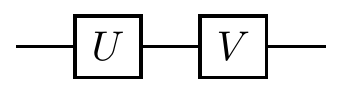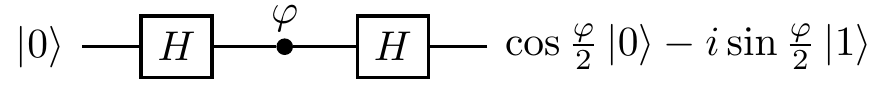## 2.7 Pauli operators

Adding to our collection of common single-qubit gates, we now look at the three Pauli operators48 \sigma_x, \sigma_y, and \sigma_z, also denoted by X, Y, and Z, respectively. These three operators, combined with the identity, satisfy a lot of nice formal properties, which we shall examine briefly here, and then return to in more detail later on, in Section 3.3.

 Identity \mathbf{1}= \begin{bmatrix}1&0\\0&1\end{bmatrix} \begin{array}{lcr}|0\rangle&\longmapsto&|0\rangle\\|1\rangle&\longmapsto&|1\rangle\end{array} Bit-flip X = \begin{bmatrix}0&1\\1&0\end{bmatrix} \begin{array}{lcr}|0\rangle&\longmapsto&|1\rangle\\|1\rangle&\longmapsto&|0\rangle\end{array} Bit-phase-flip Y = \begin{bmatrix}0&-i\\i&0\end{bmatrix} \begin{array}{lcr}|0\rangle&\longmapsto&i|1\rangle\\|1\rangle&\longmapsto&-i|0\rangle\end{array} Phase-flip Z = \begin{bmatrix}1&0\\0&-1\end{bmatrix} \begin{array}{lcr}|0\rangle&\longmapsto&|0\rangle\\|1\rangle&\longmapsto&-|1\rangle\end{array}

The identity is just a quantum wire, and we have already seen (Section 2.6) the X and Z gates as the bit-flip and phase-flip, respectively. Note that, of the X and Z gates, only the X gate has a classical analogue (namely the logical \texttt{NOT} operator). The remaining gate, the Y operator, describes the combined effect of both the bit- and the phase-flip: ZX=iY.

In fact, this is just one of the equations that the Pauli matrices satisfy. The Pauli matrices are unitary and Hermitian, they square to the identity, and they anti-commute. By this last point, we mean that \begin{aligned} XY&=-YX, \\XZ&=-ZX, \\YZ&=-ZY. \end{aligned} As already mentioned, they satisfy ZX=iY, but also any cyclic permutation of this equation (that is, replace X with Y, Y with Z, and Z with X, and repeat this as many times as you wish).

These operators are also called sigma operators (usually when we use the notation \sigma_x, \sigma_y, \sigma_z) or (when written as matrices in the standard basis, as we have done) as Pauli spin matrices. They are so ubiquitous in quantum physics that they should certainly be memorised.

### 2.7.1 From bit-flips to phase-flips, and back again

The Pauli Z gate is a special case of a phase gate P_\varphi with \varphi=\pi. When we insert it into the interference circuit we obtainIf you wish to verify this, write the Hadamard gate as H = (X+Z)/\sqrt{2} and use the properties of the Pauli operators. So the Hadamard gate turns phase-flips into bit-flips, but it also turns bit-flips into phase-flips:Let us also add, for completeness, that HYH=-Y. You will see these identities again and again, especially when we discuss quantum error corrections.49 \begin{aligned} HXH &= Z \\HZH &= X \\HYH &= -Y \end{aligned}

1. Most of the time we refer to “operators” as “matrices”, where the implicit assumption is that we are using the standard basis \{|0\rangle,|1\rangle\}.↩︎

2. Unitaries, such as H, that take the three Pauli operators to the Pauli operators via conjugation form the so-called Clifford group, which we will meet later on. Which phase gate is in the Clifford group of a single qubit?↩︎